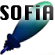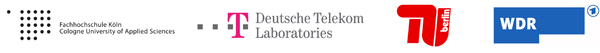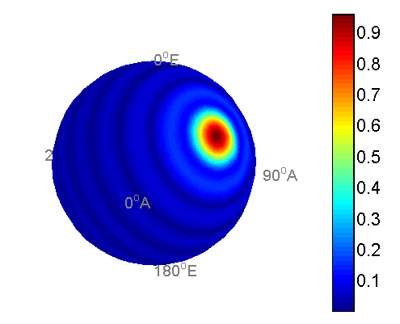Welcome to SOFiA
Who is behind SOFiA
Feature overview
System overview
Function reference
mergeArrayData
F/D/T
gauss
lebedev
S/W/G
S/T/C
W/G/C
S/F/E
M/F
R/F/I
P/D/C
I/T/C
makeMTX
makeIR
visual3D
Coordinate System
Application Examples
Example 1
Example 2
Example 3
Example 4
Example 5
Example 6
Example 7
Example 8
Array Datasets
VariSphear system
Groups and Mailinglists
Contact and Support
How to Reference

# SOFiA application example 1

In the first example we generate an ideal full audio spectrum unity plane wave of order N=9 impinging from Azimuth = pi/3 and Elevation = pi/3 to a rigid sphere array using W/G/C. We observe the array response in a single fft-bin using makeMTX and visual3D.

## File(s)

Run `sofiaAE1.m`.

## Output## Code

 ```/* % SOFiA example 1: Ideal unity plane wave simulation % SOFiA Version : R11-1220 clear all; clc; % Generate an ideal plane wave using W/G/C (Wave Generator Core);``` ``` N = 9; % Order r = 0.5; % Array radius ac = 2; % Array configuration, 2: Rigid sphere array FS = 48000; % Sampling Frequency NFFT = 128; % FFT-Bins AZ = pi/3; % Azimuth angle EL = pi/3; % Elevation angle [Pnm, kr] = sofia_wgc(N, r, ac, FS, NFFT, AZ, EL); % Make radial filters for the rigid sphere array Nrf = 9; % radial filter order dn = sofia_mf(Nrf; kr; ac); Make visualization MTX Nmtx = 9; krIndex = 30 % Here we select the kr-bin (Frequency) to display mtxData = sofia_makeMTX(Nmtx, Pnm, dn, krIndex); % Visualization with Style 0 figure(1) clf(); sofia_visual3D(mtxData, 0); */ ```